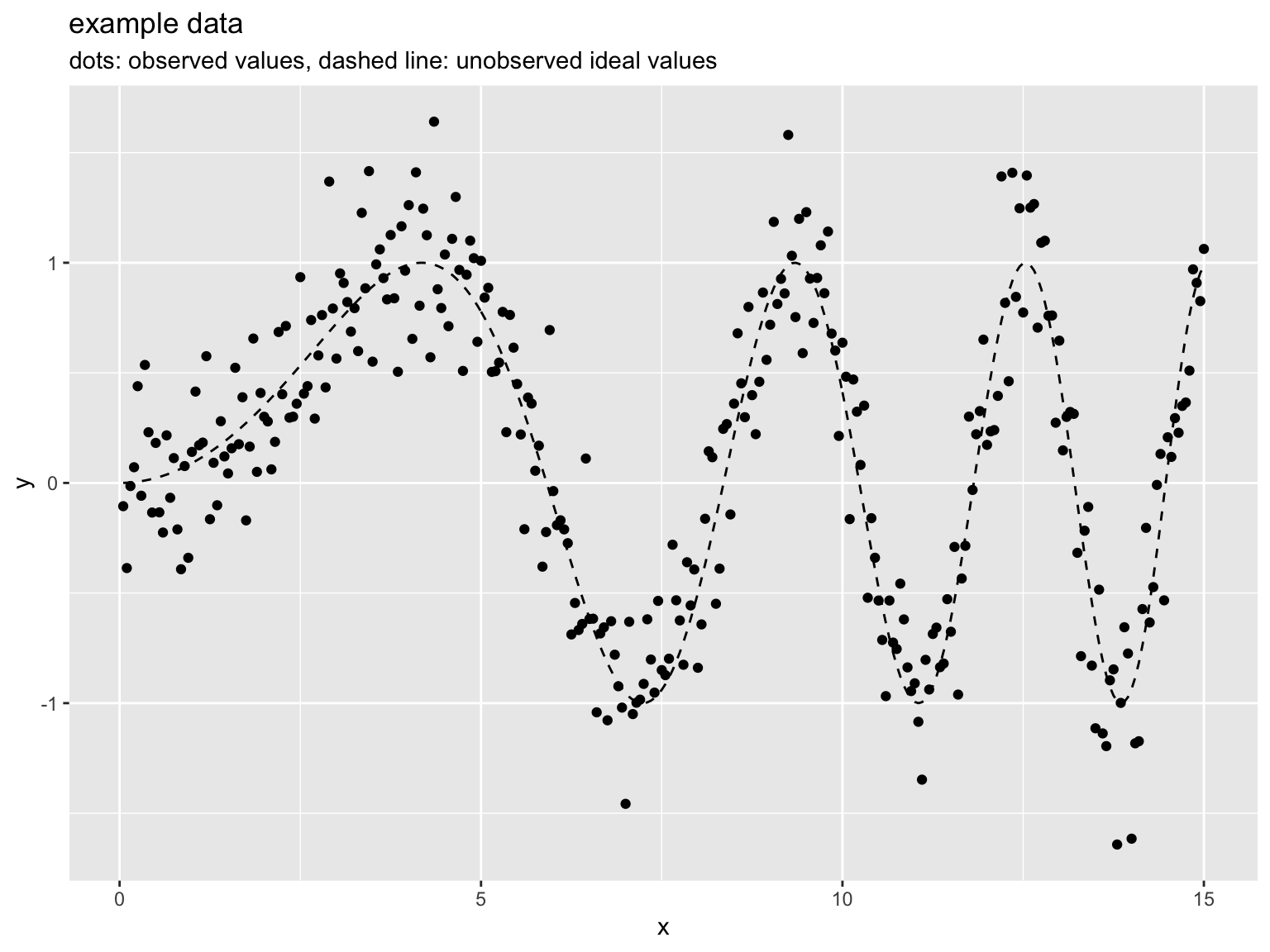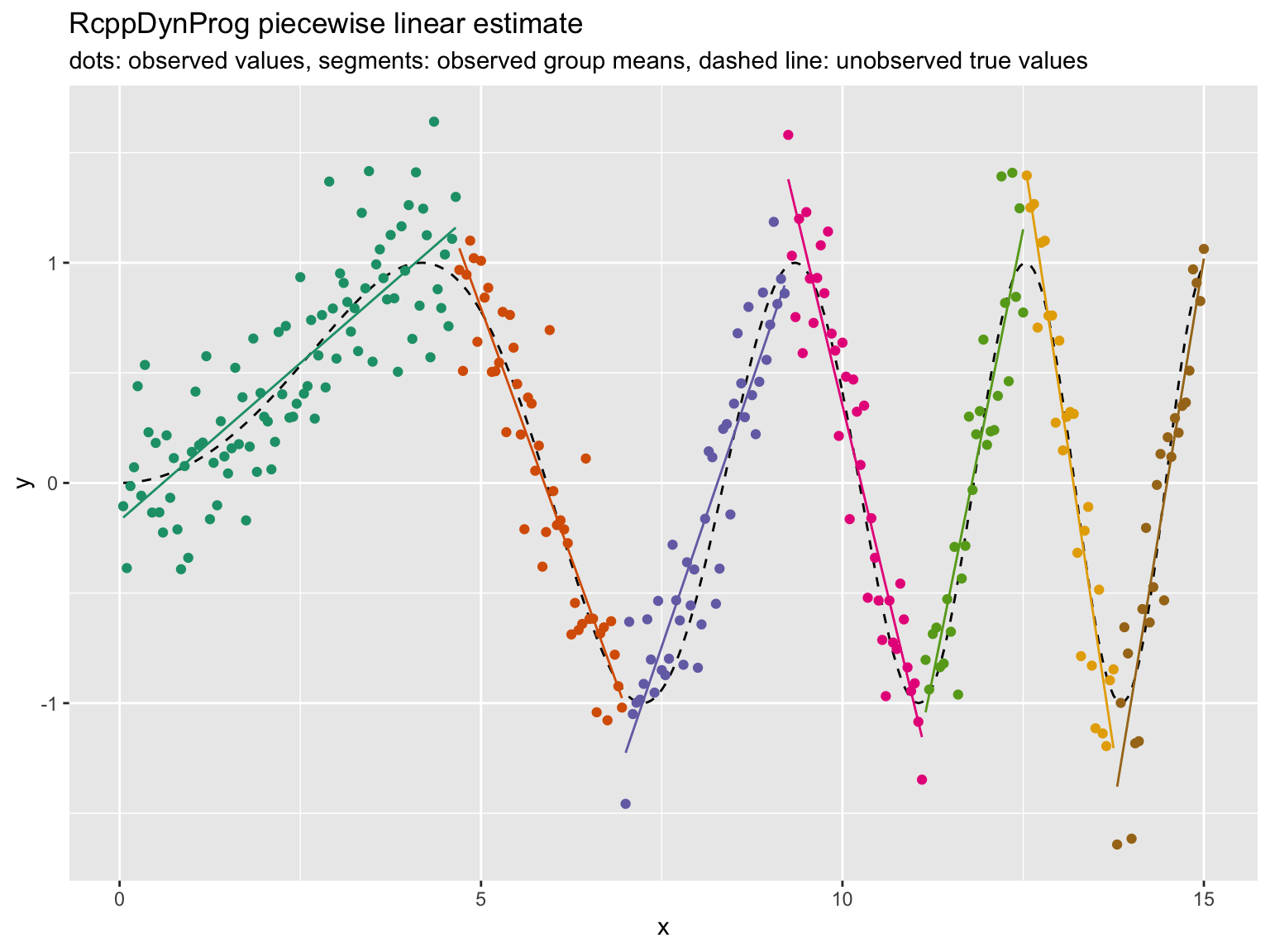# Why RcppDynProg is Written in C++

The (matter of opinion) claim:

“When the use of C++ is very limited and easy to avoid, perhaps it is the best option to do that […]”

(source discussed here)

got me thinking: does our own RcppDynProg package actually use C++ in a significant way? Could/should I port it to C? Am I informed enough to use something as complicated as C++ correctly?

RcppDynProg implements a nifty concise dynamic programming solution to a segmentation problem. It can automatically partition graphs such as the following:into the following:(details found here).

But is the package really using C++ in any significant way? The implementation is just the usual sort of index chasing needed to fill in a dynamic programming table. Looking at it superficially, the package is not doing anything deep or really using and C++ libraries in a fundamentally interesting manner.

But then it hit me: the package is indexing into arrays. With native C pointer types we would not have any bounds checking on the indexing. With the C++ classes we get bounds checking. This may seem like a small thing, but it is huge. With C pointer types if you have an out of bounds indexing error when writing a value: you may corrupt memory and that can have fairly unbounded consequences. With C++ an out of bounds indexing error causes an exception, code that executes without exception is then a proof the execution didn’t attempt out of bounds indexing.

So RcppDynProg is using C++ in a significant way: it is using it for safety guarantees on array indexing. R users expect safety guarantees on array indexing, as it is a service R supplies. So an extension package that incorporates index bounds checking can be “more R like.” This simple point makes me think many “doesn’t seem to be using C++ in any deep way” packages are also acquiring deep benefits in using C++.

Are there risks in using something as involved as a combination of R, C++, and Rcpp all at the same time for small new project?

Yes.

But I have tried to mitigate them. I have not used new/delete (used only stack-allocated C++ objects), use reference arguments (to try and minimize object construction/destruction), not defined classes with non-trivial destructors, not knowingly calling back to R functions (though I am using some Rcpp adapted data structures), and generally tried to stay in a generic tame sub-dialect of C++.

I would be happy to incorporate any polite critiques/improvements of the C++ code (found here). If there is something that is obviously wrong to an expert, I would be happy to move to what is obviously right to the experts. (Frankly the thing that most concerns me is: correctly modeling class lifetime and interaction-with/protection-from R’s garbage collector. I think I coded in a style that allows Rcpp to control these issues correctly, but I may stand to be corrected.)

Note: C++ structures such as `NumericVector` do in fact index bounds check if you use `()` notation instead of `[]` notation. RcppDynProg tries to use `()` throughout to get the index bounds checking. Below is a quick example of the difference.

```library("Rcpp")

f_good <- cppFunction('NumericVector oob(NumericVector x) {
int n = x.size();
if(n>0) {
x(0) = 5.0; // in bounds
}
return x;
}')

f_good(c(1, 2))
#  5 2

f_bad1 <- cppFunction('NumericVector oob(NumericVector x) {
int n = x.size();
x(n+10) = 5.0; // out of bounds, checked
return x;
}')

# Error in f_bad1(c(1, 2)) : Index out of bounds: [index=12; extent=2].

f_bad2 <- cppFunction('NumericVector oob(NumericVector x) {
int n = x.size();
x[n+10] = 5.0; // out of bounds, not checked- memory corruption
return x;
}')